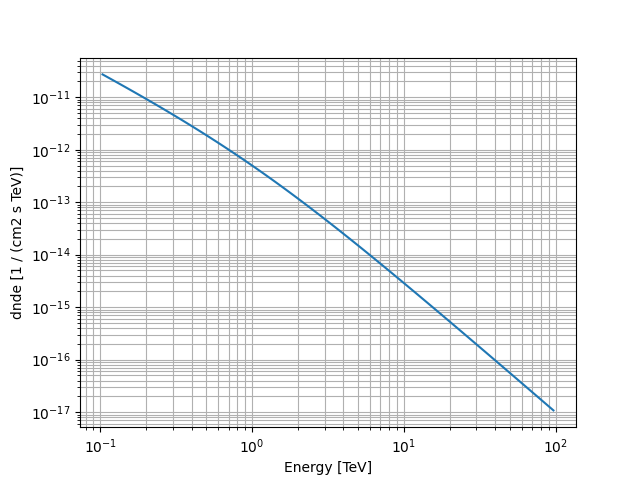# Smooth broken power law spectral model#

This model parametrises a smooth broken power law spectrum.

It is defined by the following equation:

$\phi(E) = \phi_0 \cdot \left( \frac{E}{E_0} \right)^{-\Gamma1}\left(1 + \frac{E}{E_{break}}^{\frac{\Gamma2-\Gamma1}{\beta}} \right)^{-\beta}$

## Example plot#

Here is an example plot of the model:

from astropy import units as u
import matplotlib.pyplot as plt
from gammapy.modeling.models import Models, SkyModel, SmoothBrokenPowerLawSpectralModel

energy_bounds = [0.1, 100] * u.TeV
model = SmoothBrokenPowerLawSpectralModel(
index1=1.5,
index2=2.5,
amplitude="1e-12 TeV-1 cm-2 s-1",
ebreak="1 TeV",
reference="1 TeV",
beta=1,
)
model.plot(energy_bounds)
plt.grid(which="both")## YAML representation#

Here is an example YAML file using the model:

model = SkyModel(spectral_model=model, name="smooth-broken-power-law-model")
models = Models([model])

print(models.to_yaml())

components:
-   name: smooth-broken-power-law-model
type: SkyModel
spectral:
type: SmoothBrokenPowerLawSpectralModel
parameters:
-   name: index1
value: 1.5
-   name: index2
value: 2.5
-   name: amplitude
value: 1.0e-12
unit: cm-2 s-1 TeV-1
-   name: ebreak
value: 1.0
unit: TeV
-   name: reference
value: 1.0
unit: TeV
-   name: beta
value: 1.0


Gallery generated by Sphinx-Gallery